# Different Ways For Integer to String Conversions in Java

• Difficulty Level : Easy
• Last Updated : 10 Jun, 2022

We generally counter with such conversion articles because many operations can be performed over a string while we are limited to when it comes to integers. We have a wide varied list of in-built method in the String class that helps us perform hassle-free operations.
Suppose we are required to concatenate two integers then it would become a tedious job as we need to go through as we need to deal with the number system corresponding to which we will be playing mathematics within the number system. But in Java, in order to convert integer to string, we have some inbuilt methods and classes which make out work too easy.

Tip: We generally convert primitive class data members types though we have the concept of Wrapper classes to Strings because in practical programming in java we deal with strings.

### Different Methods For Integer to String Conversions

1. Using toString() method of Integer class
2. Using valueOf() method of String class
3. Using Integer(int).toString() method of Integer class
4. Using DecimalFormat Class
5. Using StringBuffer class
6. using StringBuilder class
8. Using concatenation with an empty string

### Method 1: Using toString Method of Integer Class

The Integer class has a static method that returns a String object representing the specified int parameter. The argument is converted and returned as a string instance. If the number is negative, the sign will be preserved.

Example:

## Java

 `// Java Program to Illustrate Integer to String Conversions``// Using toString() Method of Integer Class` `// Main class``class` `GFG {` `    ``// Main driver method``    ``public` `static` `void` `main(String args[])``    ``{``        ``// Custom input integers``        ``int` `a = ``1234``;``        ``int` `b = -``1234``;` `        ``// Converting integer to string``        ``// using toString() method``        ``String str1 = Integer.toString(a);``        ``String str2 = Integer.toString(b);` `        ``// Printing the above strings that``        ``// holds integer``        ``System.out.println(``"String str1 = "` `+ str1);``        ``System.out.println(``"String str2 = "` `+ str2);``    ``}``}`

Output

```String str1 = 1234
String str2 = -1234```

Example A:

## Java

 `// Java Program to Illustrate Integer to String Conversions``// Using valueOf() Method of String class` `// Main class``class` `GFG {` `    ``// Main driver method``    ``public` `static` `void` `main(String args[])``    ``{``        ``// Custom integer input``        ``int` `c = ``1234``;` `        ``// Converting above integer to string``        ``// using valueOf() Method``        ``String str3 = String.valueOf(c);` `        ``// Printing the integer stored in above string``        ``System.out.println(``"String str3 = "` `+ str3);``    ``}``}`

Output

`String str3 = 1234`

Example B:

## Java

 `// Java Program to Illustrate Integer to String Conversions``// Using valueOf() Method of String class` `// Main class``class` `GFG {``  ` `    ``// Main driver method``    ``public` `static` `void` `main(String args[])``    ``{``        ``// Passing input integer as in argument and``        ``// storing it in a string``        ``String str3 = String.valueOf(``1234``);` `        ``// Printing the integer stored in above string``        ``System.out.println(``"String str3 = "` `+ str3);``    ``}``}`

Output

`String str3 = 1234`

### Method 3: Using toString() method of the Integer class

It is different from method 1 as proposed above, as in this method we use an instance of the Integer class to invoke its toString() method.

Example A:

## Java

 `// Java Program to Illustrate``// Integer to String Conversions``// Using Integer(int).toString() Method` `// Importing required classes``import` `java.util.*;` `// Main class``class` `GFG {``  ` `    ``// Main driver method``    ``public` `static` `void` `main(String args[])``    ``{``        ``// Custom input integer``        ``int` `d = ``1234``;` `        ``// Creating an object of Integer class``        ``// inside main() method``        ``Integer obj = ``new` `Integer(d);``        ``String str4 = obj.toString();` `        ``// Printing the above string``        ``// holding integer value``        ``System.out.println(``"String str4 = "` `+ str4);``    ``}``}`

Output: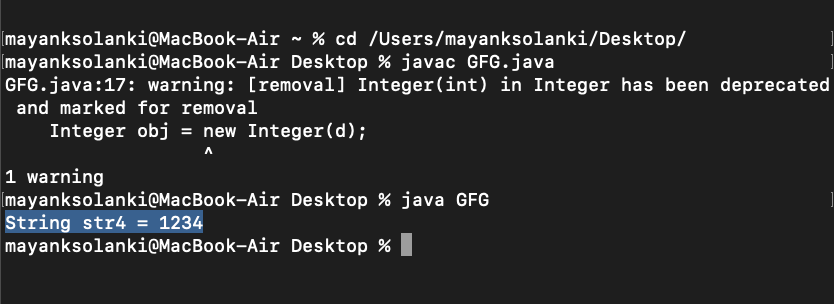Example B:

## Java

 `// Java Program to Illustrate``// Integer to String Conversions``// Using toString() Method of``// Integer Class` `// Importing required classes``import` `java.util.*;` `// Main class``class` `GFG {` `    ``// Main driver method``    ``public` `static` `void` `main(String args[])``    ``{``        ``// Custom input integer``        ``int` `d = ``1234``;` `        ``// Converting integer to string``        ``// using toStirng() method of Integer class``        ``String str4 = ``new` `Integer(d).toString();` `        ``// Printing the integer value stored in above string``        ``System.out.println(``"String str4 = "` `+ str4);``    ``}``}`

Output: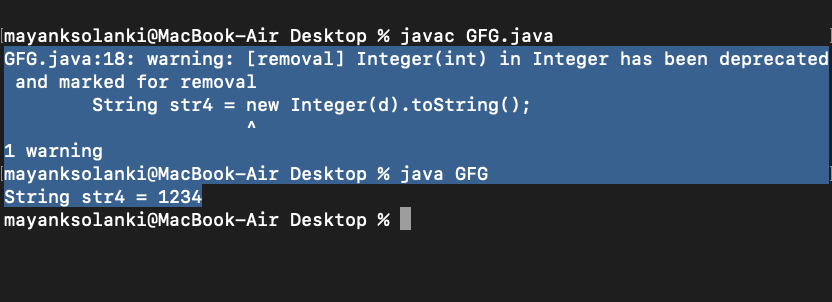Example C:

## Java

 `// Java Program to Illustrate``// Integer to String Conversions``// Using toString() Method of``// Integer Class` `// Importing required classes``import` `java.util.*;` `// Main class``class` `GFG {` `    ``// Main driver method``    ``public` `static` `void` `main(String args[])``    ``{``        ``// Passing integer inside object as soon it is``        ``// created via parametrised constructor and``        ``// converting integer to string using toString()``        ``// method and storing it into a String``        ``String str4 = ``new` `Integer(``1234``).toString();` `        ``// Printing the above string holding integer value``        ``System.out.println(``"String str4 = "` `+ str4);``    ``}``}`

Output: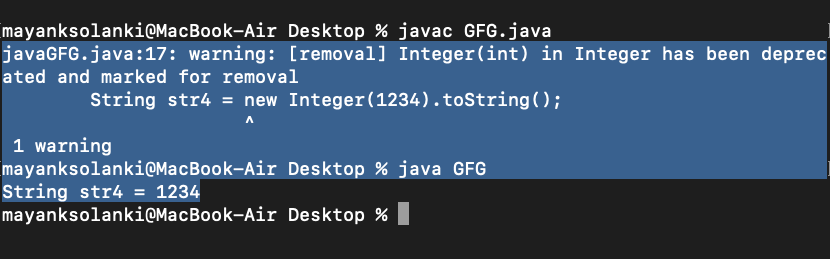Output explanation: If the variable is of primitive type (int), it is better to use Integer.toString(int) or String.valueOf(int). But if the variable is already an instance of Integer (wrapper class of the primitive type int), it is better to just invoke its toString() method as shown above.

Note: This method is not efficient as an instance of the Integer class is created before conversion is performed.

### Method 4: Using DecimalFormat Class

DecimalFormat is a class that formats a number to a String.

Example A:

## Java

 `// Java Program to Illustrate Integer to String Conversions``// Using DecimalFormat Class` `// Importing required classes``import` `java.text.DecimalFormat;` `// Main class``class` `GFG {` `    ``// Main driver method``    ``public` `static` `void` `main(String args[])``    ``{``        ``// Custom integer input``        ``int` `e = ``12345``;` `        ``// Creating an object of DecimalFormat class``        ``// inside main() method``        ``DecimalFormat df = ``new` `DecimalFormat(``"#"``);` `        ``// Formatting the integer to string``        ``// and storing it in a string``        ``String str5 = df.format(e);` `        ``// Printing the above stored value``        ``// inside a string``        ``System.out.println(str5);``    ``}``}`

Output

`12345`

Example B:

## Java

 `// Java Program to Illustrate``// Integer to String Conversions``// Using DecimalFormat Class` `// Importing required classes``import` `java.text.DecimalFormat;` `// Main class``class` `GFG {` `    ``// Main driver method``    ``public` `static` `void` `main(String args[])``    ``{``        ``// Input integer value``        ``int` `e = ``12345``;` `        ``// Creating an object of DecimalFormat class``        ``// inside main() method``        ``DecimalFormat df = ``new` `DecimalFormat(``"#,###"``);` `        ``// Converting above integral value to string``        ``String Str5 = df.format(e);` `        ``// Printing the value stored in above string``        ``System.out.println(Str5);``    ``}``}`

Output: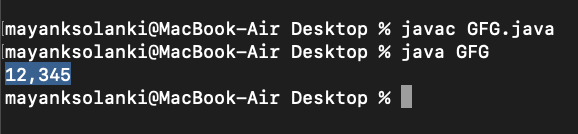Tip: Using this method, you can specify the number of decimal places and comma separator for readability.

### Method 5: Using StringBuffer class

StringBuffer is a class that is used to concatenate multiple values into a String.

Example A:

## Java

 `// Java Program to Illustrate``// Integer to String Conversions``// Using StringBuffer Class` `// Main class``class` `GFG {``  ` `    ``// Main driver method``    ``public` `static` `void` `main(String args[])``    ``{``        ``// Integer input value``        ``int` `f = ``1234``;` `        ``// Creating an object of StringBuffer class``        ``StringBuffer sb = ``new` `StringBuffer();``        ``sb.append(f);` `        ``String str6 = sb.toString();` `        ``System.out.println(``"String str6 = "` `+ str6);``    ``}``}`

Output

`String str6 = 1234`

Example B:

## Java

 `// Java Program to Illustrate``// Integer to String Conversions``// Using StringBuffer Class` `// Main class``class` `GFG {` `    ``// Main driver method``    ``public` `static` `void` `main(String args[])``    ``{``        ``String str6``            ``= ``new` `StringBuffer().append(``1234``).toString();` `        ``System.out.println(``"String str6 = "` `+ str6);``    ``}``}`

Output:

`String str6 = 1234`

### Method 6: Using StringBuilder Class

StringBuilder works similarly but is not thread-safe like StringBuffer.

Example A:

## Java

 `// Java Program to Illustrate``// Integer to String Conversions``// Using StringBuilder Class` `// Main class``class` `GFG {` `    ``// Main driver method``    ``public` `static` `void` `main(String args[])``    ``{``        ``// Input integer``        ``int` `g = ``1234``;` `        ``// Creating an object of StringBuilder class``        ``// inside main() method``        ``StringBuilder sb = ``new` `StringBuilder();``        ``sb.append(g);` `        ``String str7 = sb.toString();` `        ``// Printing the value stored in above string``        ``System.out.println(``"String str7 = "` `+ str7);``    ``}``}`

Output

`String str7 = 1234`

Example B:

## Java

 `// Java Program to Illustrate Different Ways for``// Integer to String Conversions``// Using StringBuilder Class` `// Main class``class` `GFG {` `    ``// Main driver method``    ``public` `static` `void` `main(String args[])``    ``{``        ``String str7``            ``= ``new` `StringBuilder().append(``1234``).toString();` `        ``// Printing the value stored in above string``        ``System.out.println(``"String str7 = "` `+ str7);``    ``}``}`

Output

`String str7 = 1234`

Note: All the examples above use the base (radix) 10. Following are convenient methods to convert to binary, octal, and hexadecimal systems. The arbitrary custom number system is also supported.

Example: Binary

## Java

 `// Java Program to Illustrate Integer to String Conversions``// Using Special Radix In Binary Numbers` `// Main class``class` `GFG {` `    ``// Main driver method``    ``public` `static` `void` `main(String args[])``    ``{``        ``// Input integer``        ``int` `h = ``255``;``        ``String binaryString = Integer.toBinaryString(h);` `        ``// Printing the binary number stored in above string``        ``System.out.println(binaryString);``    ``}``}`

Output

`11111111`

Output Explanation: 11111111 is the binary representation of the number 255.

Example: Octal

## Java

 `// Java Program to Illustrate Integer to String Conversions``// Using Special Radix In Octal Numbers` `// Main class``class` `GFG {` `    ``// Main driver method``    ``public` `static` `void` `main(String args[])``    ``{``        ``// Custom input integer``        ``int` `i = ``255``;``        ``String octalString = Integer.toOctalString(i);` `        ``// Printing the octal number stored in above string``        ``System.out.println(octalString);``    ``}``}`

Output

`377`

377 is the octal representation of the number 255.

## Java

 `// Java Program to Illustrate Integer to String Conversions``// Using Special Radix In Hexadecimal Numbers` `// Main class``class` `GFG {` `    ``// Main driver method``    ``public` `static` `void` `main(String args[])``    ``{``        ``// Custom input integer``        ``int` `j = ``255``;``        ``String hexString = Integer.toHexString(j);` `        ``// Printing the hexadecimal number``        ``// stored in above string``        ``System.out.println(hexString);``    ``}``}`

Output

`ff`

The ff is the hexadecimal representation of the number 255.

Approach: We are using the toString() method of the Integer class to get it converted into a string where additionally we will be passing a value as an argument known as radix. One can use any other custom base/radix when converting an int to string. In the below example, for illustration purposes, we are considering the base 7 number system.

Example:

## Java

 `// Java Program to Illustrate Integer to String Conversions``// Using Custom Radix` `// Main class``class` `GFG {` `    ``// Main driver method``    ``public` `static` `void` `main(String args[])``    ``{``        ``// Input integer value``        ``int` `k = ``255``;` `        ``// Setting base as 7, converting integer to string``        ``// using toString() method and``        ``// storing it into a string``        ``String customString = Integer.toString(k, ``7``);` `        ``// Printing value stored in above string``        ``System.out.println(customString);``    ``}``}`

Output: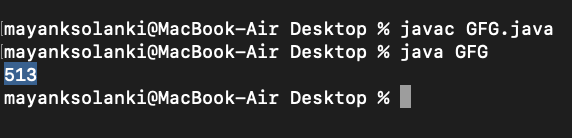513 is the representation of the number 255 when written in the base 7 system.

### Method 8: Using concatenation with an empty string

Approach: Here we will declare an empty string and using the ‘+’ operator, we will simply store the resultant as a string. Now by virtue of this, we are successfully able to append and concatenate these strings.

Example:

## Java

 `// Java Program to Illustrate Integer to String Conversions``// Using Concatenation with Empty String` `// Main class``class` `GFG {` `    ``// Main driver method``    ``public` `static` `void` `main(String args[])``    ``{``        ``// Custom integer values``        ``int` `a = ``1234``;``        ``int` `b = -``1234``;` `        ``// Concatenating with empty strings``        ``String str1 = ``""` `+ a;``        ``String str2 = ``""` `+ b;` `        ``// Printing the concatenated strings``        ``System.out.println(``"String str1 = "` `+ str1);``        ``System.out.println(``"String str2 = "` `+ str2);``    ``}``}`

Output

```String str1 = 1234
String str2 = -1234```